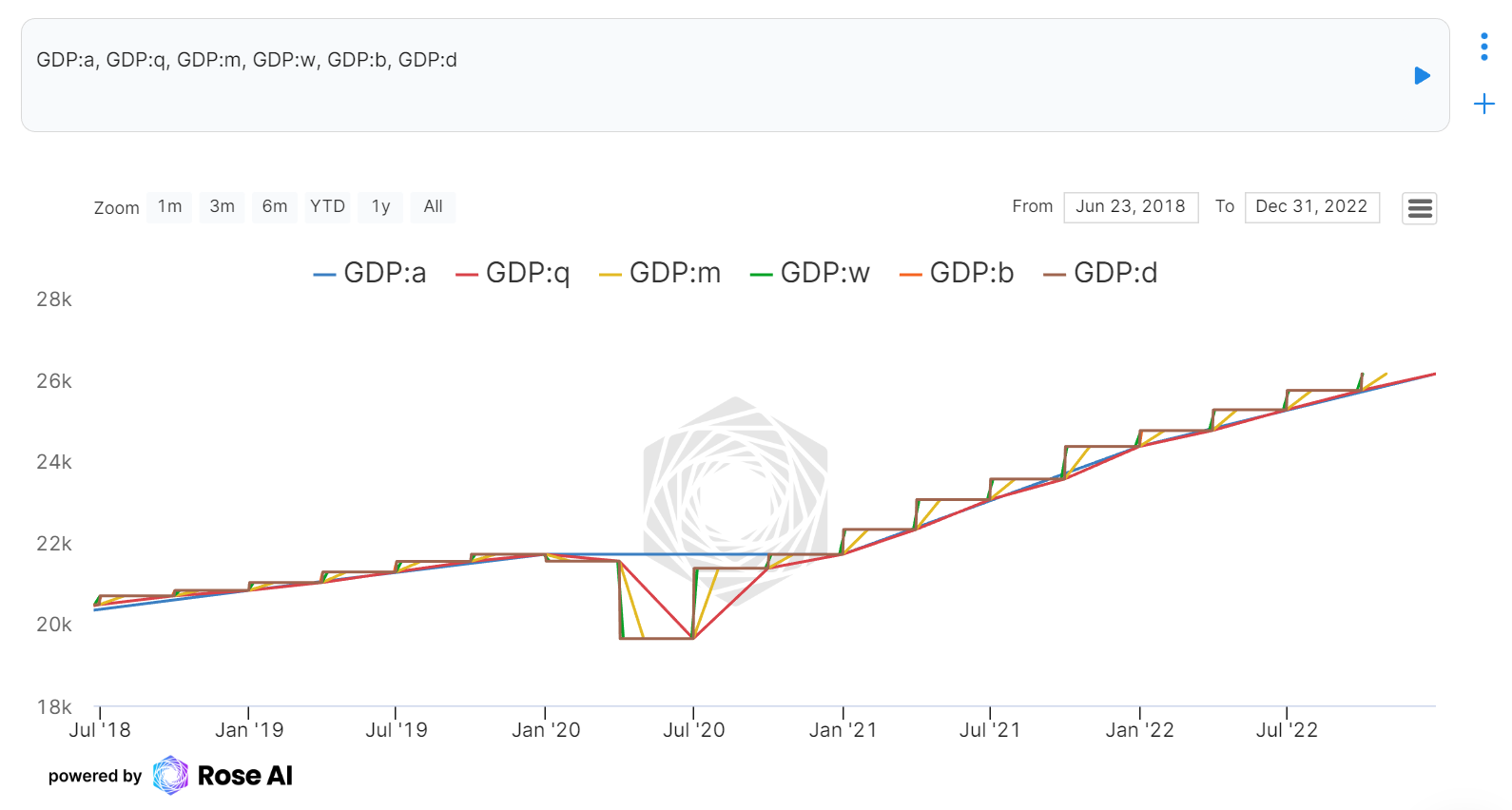# Using Transformations

There are over 100 transformations in Rose to help in your analysis. Transformations in Rose follow a left-to-right order of operations. As we work to build out our documentation, please refer to the Transformations Specifications page for a list of all the transformations, their parameters and descriptions.

Try running this in a code block :material-code-tags::

``````functions
``````## How to Call Transformations in Rose

The basic syntax for calling a transformation in Rose is:

rosecode + : + transformation name + (optional parameters)

Example: To view the year-over-year change of the GDP in the USA, you would run the following, both will return the same result.

``````usa.gdp:yoy
``````

OR

``````usa.gdp:yoy(geo)
``````

The default parameter for the `:yoy()` transformation is geometric/geo change; other options include arithmetic/ari, level/lvl or percent/pct change. Investopedia: The Difference Between the Arithmetic Mean and Geometric Mean

## Basic Transformations

### Simple Math Transformations

Operation Operator Rose Transformation
subtraction - :sub
multiplication * :mult
division / :div
power ^ :power
absolute value | x | :abs

### Intermediate Math Transformations

Operation Description Rose Transformation
mean sum of values divided by count of values :mean
median center value of sorted values :median
standard deviation standard deviation of a timeseries :std
z-score z-score of a timeseries where
z = ( x - mean ) / std
:z
flip multiplies timeseries by -1 :flip
inverse ( 1 / x ) of a timeseries :inv
log natural log of a timeseries :log
returns geometric ( / ) change of a timeseries :returns

Operation Description Rose Transformation
correlation correlation between two timeseries :corr
cumulative product cumulative product ( * ) of a timeseries :cum
cumulative sum cumulative sum ( + ) of a timeseries :cumsum

### Update vs Refresh

Two important transformations to be aware of in Rose are `:update` and `:refresh`. Both are used to get the latest data on a rosecode, but whereas `:refresh` is an internal cache-clearing transformation, `:update` is an external database call (example: to get the latest data from FRED).

## Chaining and Nesting Transformations

Transformations are useful on their own but when used together they are powerful!

### Chaining Transformations

Chaining transformations in Rose follows a left-to-right order of operations.

Example:

Let's say you want the annual value of the GDP but the original data is in billions of dollars and reported monthly. By chaining the `:mult` (multiply) and `:a` (resample annually) transformations, we can get our desired format.

``````GDP:mult(1000000000):a
``````### Nesting Transformations

Similar to chaining, transformations can also be nested using sets of paranthesis ( ). This is useful when using a timeseries as a parameter in another transformation.

Example:

If we want to calculate the GDP per capita, we would also want to divide the population, but it is important that both are in the same frequency. In the below exmaple, the `:mult` (multiply) and `:a` (resample annually) is nested inside the `:div` (divide) transformation.

``````GDP:mult(1000000000):a:div(usa.pop:a)
``````## Resampling Transformations

Resampling data across frequencies can be painful and frustrating. To make this easier, Rose has a built in resample transformation, simplifying the conversion between daily (business days, all days), weekly, monthly, quarterly, and annual data. Also see the `:resample` transformation

Resample Frequency Rose Transformation
(skips weekends and holidays)
:b
days :d
weekly :w
monthly :m
quarterly :q
annually / yearly :a

Resampling your data can yield vastly different results. Let's view the same timeseries resampled to each of these intervals.## Map Transformations

Maps, our term for tables, provide flexibility within Rose outside of timeseries and allow for complex transformations. Here are some of the most commonly used transformations for maps. More Map Transformations

Operation Description Rose Transformation
filter filters a map by a given condition ex: =, >, >=... :filter
columns returns a map of the column names in the given map :columns
limit limits the number of rows in a map to either a number of rows or a percentage of rows :limit
mean returns the mean of a column, can be used on a column of rosecodes to create a mean timeseries of the column :mean
search returns any row in a map that contains the search term :search
sort sorts a map based on the given column :sort
to timeseries converts a map to a timeseries (map must be a column of dates and a column of values) :ts